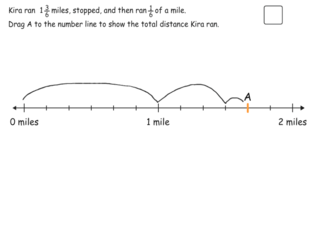# Interactive Problem Bank

•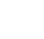Fraction Circles
•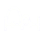Number Lines
•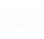Fraction Bars
•Sets
•Area Models
•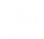Base Ten Blocks

Students add and subtract fractions and mixed numbers with like denominators using fraction circles, fraction bars, and real-world contexts.

### Adding Fractions with Like Denominators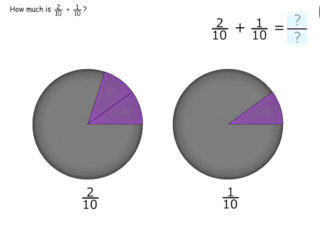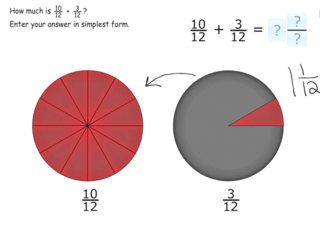### Sums Greater Than 1 Using Models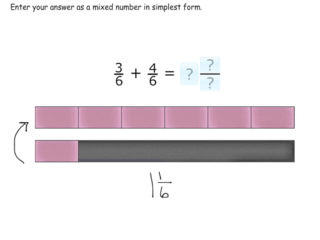### Subtracting Fractions with Like Denominators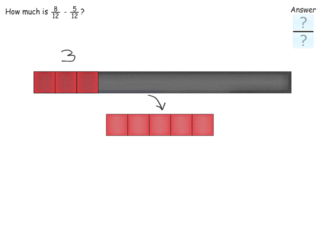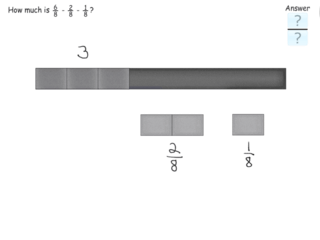### Adding Whole Numbers and Fractions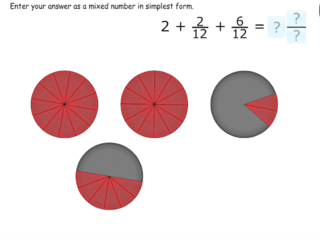### Adding Mixed Numbers and Fractions Using Models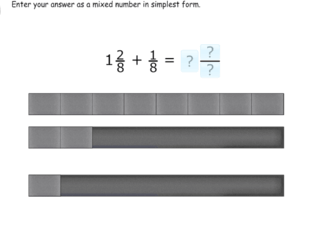### Subtracting Fractions from Mixed Numbers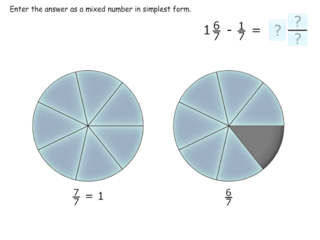### Sums Greater Than 1 Using Number Line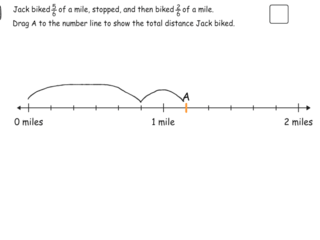### Adding Mixed Numbers and Fractions Using Number Line